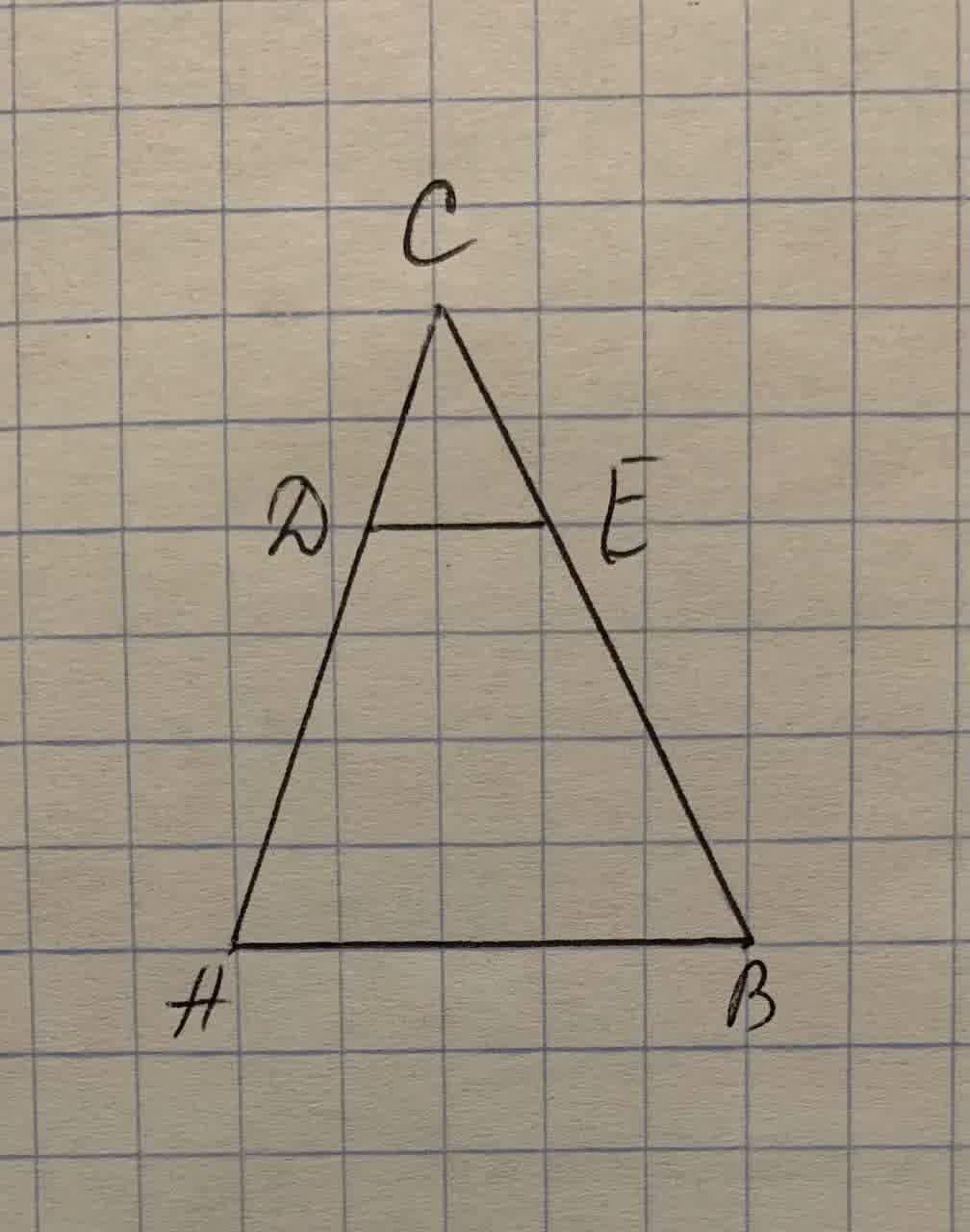## Prove that if a line is drawn parallel to the base of a triangle, it cuts off a triangle similar to the given triangle.

TrigonometryProve that if a line is drawn parallel to the base of a triangle, it cuts off a triangle similar to the given triangle.9 months ago
Prove that if a line is drawn parallel to the base of a triangle, it cuts off a triangle similar to the given triangle.Given: △ ABC,DEparallel AB Prove:△ DEC~△ ABC